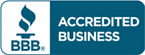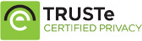# Solution manaual for Spreadsheet Modeling and Decision Analysis 6th Edition by Cliff Ragsdale

\$30.00

Solution manaual
Answers key  with excel Spreadsheet Modeling and Decision Analysis 6th Edition by Cliff Ragsdale## Description

Solution manaual for Spreadsheet Modeling and Decision Analysis 6th Edition by Cliff Ragsdale

1. Introduction to Modeling and Decision Analysis.
2. Introduction to Optimization and Linear Programming.
3. Modeling and Solving LP Problems in a Spreadsheet.
4. Sensitivity Analysis and the Simplex Method.
5. Network Modeling.
6. Integer Linear Programming.
7. Goal Programming and Multiple Objective Optimization.
8. Nonlinear Programming & Evolutionary Optimization.
9. Regression Analysis.
10. Discriminant Analysis.
11. Time Series Forecasting.
12. Introduction to Simulation using Risk Solver Platform.
13. Queuing Theory.
14. Decision Analysis.
15. Project Management (online)

## Reviews

There are no reviews yet.